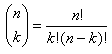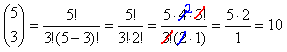Pascal's Triangle

There is a unique pattern of numbers known as Pascal’s triangle. This pattern has applications in many areas of mathematics including combinatorics. To explore many of the attributes of Pascal’s Triangle select the following link, paying close attention to the text about combinations:

Pascal's Triangle

To find any entry in Pascal’s Triangle apply the “n choose k” formula:For example, in row 5, term 3 is 10. Use the formula to verify: